# DAV Class 5 Maths Chapter 4 Worksheet 12 Solutions

The DAV Class 5 Maths Book Solutions Pdf and DAV Class 5 Maths Chapter 4 Worksheet 12 Solutions of Fractional Numbers offer comprehensive answers to textbook questions.

## DAV Class 5 Maths Ch 4 Worksheet 12 Solutions

Question 1.
Fill in the blanks.
(a)Solution:(b)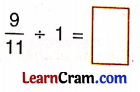Solution: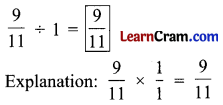(c)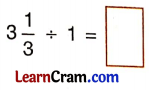Solution: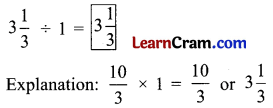(d)Solution: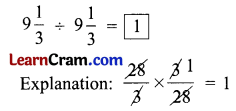(e)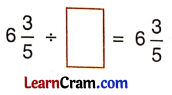Solution:Explanation: A fraction divided by one is fraction itself.

(f)Solution: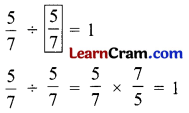(g)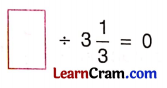Solution: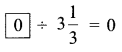Explanation: Zero divided by any fraction is zero.

(h)Solution:Explanation: Zero divided by any fraction is zero.

(i)Solution: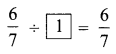Explanation: A fraction divided by one is a fraction itself.

DAV Class 5 Maths Chapter 4 Worksheet 12 Notes

I. Division of a fractional number by 1.
A fraction divided by one is the fraction itself.

Example 1.
Find $$\frac{2}{7}$$ ÷ 1
Solution:
$$\frac{2}{7}$$ ÷ 1
= $$\frac{2}{7} \times \frac{1}{1}$$ (Multiplying by reciprocal of one that is one itself)
= $$\frac{2}{7}$$II. Division of 0 by a fractional number.
Zero divided by any fraction is zero.

Example 2.
Find is 0 ÷ $$\frac{2}{3}$$
Solution:
0 ÷ $$\frac{2}{3}$$
= 0 × $$\frac{3}{2}$$ (Multiplying by the reciprocal of $$\frac{2}{3}$$)
= 0

III. Division of a fractional number by itself.
A fraction divided by itself is one.

Example 3.
Find $$\frac{3}{7} \div \frac{3}{7}$$
Solution:
$$\frac{3}{7} \div \frac{3}{7}$$
= $$\frac{3}{7}$$ × $$\frac{7}{3}$$ (Multiplying by reciprocal of $$\frac{3}{7}$$)
= 1